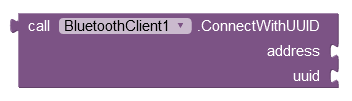# Getting an error 507: unable to connect to device, are you sure it is on

The robot I have works with 'Bluetooth Electronic app'. I wanted to make my own app so that I could add a web cam to the robot. But i keep getting a 507 error code. Below is my code for the app.

I am confused about why it works on the Bluetooth Electronic app but not the app I created.

This is my raspberry pi python code.
import bluetooth #-------> import bluetooth module
import RPi.GPIO as GPIO # ------> Import GPIO module so that we can controll pins

GPIO.setwarnings(False) #-------> To disable warnings
GPIO.setmode(GPIO.BOARD) #-------> GPIO pins scheme (here its set to physical board)

GPIO.setup(8, GPIO.OUT, initial=GPIO.LOW) # ------v
GPIO.setup(10, GPIO.OUT, initial=GPIO.LOW) # ---->Declaring motor1 pins(8,10) as output and low at first

GPIO.setup(3, GPIO.OUT, initial=GPIO.LOW) # -------v
GPIO.setup(5, GPIO.OUT, initial=GPIO.LOW) # ----->Declaring motor1 pins(8,5) as output and low at first

GPIO.setup(13, GPIO.OUT, initial=GPIO.LOW) #------>Declaring buzzer pin 13 as output and low at start
GPIO.setup(15, GPIO.OUT, initial=GPIO.LOW) #--------> declaring pin 15(led pin) as output and low at first

a = 0 #----> varible for storing value 1 or 0 for our led to turn on or off

server_socket=bluetooth.BluetoothSocket(bluetooth.RFCOMM) #-----> declaring our bluetooth server socket
port = 1 #-----> a variable to store value of port
server_socket.bind(("",port)) #----> bindind port to our sever socket
server_socket.listen(1) #------>make our bluetooth sever to listen for 1 connection at a time
client_socket,address = server_socket.accept() #----> accept connection from client and get the address
print ("Accepted connection from ",address) #------> print the bluetooth address of the connected client or the device

def left():
print (" LEFT")
GPIO.output(8, GPIO.HIGH) #-----> turning left
GPIO.output(10, GPIO.LOW)
GPIO.output(3, GPIO.LOW)
GPIO.output(5, GPIO.HIGH)

def right():
print ("right")
GPIO.output(8, GPIO.LOW)
GPIO.output(10, GPIO.HIGH) #-----> turning right
GPIO.output(3, GPIO.HIGH)
GPIO.output(5, GPIO.LOW)

def forward():
print ("FORWARD")
GPIO.output(8, GPIO.HIGH)
GPIO.output(10, GPIO.LOW) #-----> move forward
GPIO.output(3, GPIO.HIGH)
GPIO.output(5, GPIO.LOW)

def back():
print ("BACKWARDS")
GPIO.output(10, GPIO.HIGH)
GPIO.output(8, GPIO.LOW)
GPIO.output(5, GPIO.HIGH) #-----> move backwards
GPIO.output(3, GPIO.LOW)

def stop():
print ("STOP")
GPIO.output(8, GPIO.LOW)
GPIO.output(10, GPIO.LOW) #-----> stop
GPIO.output(3, GPIO.LOW)
GPIO.output(5, GPIO.LOW)

data=""
while True: #------> run the below functions in loop

``````data= client_socket.recv(1024)           #-----> declaring variable "data" as the data received from the client
data = data.decode('UTF-8')              #-----> the data recieved will be in the form of byes
#         so we will convert it into strings

if (data == "F"):                        #----> if the data is f
forward()                            # then function forward

elif (data == "L"):                      #----> if the data is L
left()                               # then function left

elif (data == "R"):                      #----> if the data is R
right()                              # then function right

elif (data == "B"):                      #----> if the data is B
back()                               # then function back

elif (data == "s"):                      #----> if the data is s
stop()                               # then function stop

elif(data == "X"):                       #----> if the data is X
GPIO.output(13, GPIO.HIGH)           # then set buzzer pin 13 value high
elif(data == "x"):                       #----> if the data is x
GPIO.output(13, GPIO.LOW)            # then set buzzer pin 13 value low

elif (data == "l"):                      #----> if the data is L
a+=1                                # then increase value of variable a by 1
if(a == 2):                              #-----> if variable a value is 2 then set it back to zero
a = 0                                # then set  variable a to 0
if(a == 1):                              #------->if a value is 1
GPIO.output(15, GPIO.HIGH)           # then set led pin 15 value to High to turn on the light
if(a == 0):                              #------->if a value is 0
GPIO.output(15, GPIO.LOW)            # then set led pin 15 value to LOW to turn off the light``````I think you may get into trouble trying to send with touch-down/touch-up button events. I suggest using a ListView (or HTML Table) coupled with a send Procedure. Also, immediately before a BT send, you should check you have a connection.

It worked for me with simpler code.

Note that you will get a 507 if the Robot's Bluetooth is not switched on.

Example Blocks for the buttons:

RobotControl.aia (3.0 KB)

Example Project requesting Bluetooth Connection on SmartPhone (Android Device). It uses a Location Sensor to trigger Location Permission (may or may not be required depending on your Android version).

BT_Basic_Setup_Send.aia (7.2 KB)